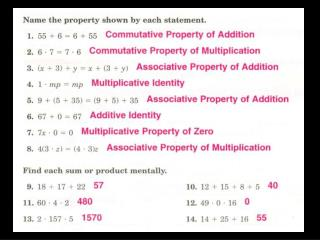# Sections 2.1, 2.2 - PowerPoint PPT PresentationDownload PresentationSections 2.1, 2.2

Sections 2.1, 2.2
Download Presentation## Sections 2.1, 2.2

- - - - - - - - - - - - - - - - - - - - - - - - - - - E N D - - - - - - - - - - - - - - - - - - - - - - - - - - -
##### Presentation Transcript

1. Sections 2.1, 2.2 Positive and Negative Numbers (Part 1)

2. What are integers? The whole numbers and their opposites. …-3, -2, -1, 0, 1, 2, 3… (Numbers marked on a standard number line.)

3. Graph the following integers. -5, -1, 10, 4, -9

4. Graph the following integers. -5, -1, 10, 4, -9 What do the following symbols mean? <, >, =

5. What Is Absolute Value? The distance a number is from zero.

6. Example 1-4d Evaluate each expression.

7. Example 1-4d Evaluate each expression. Let x = 3, y = 12, z = -9

8. 4 -  you could teach it right now 3 – you could ace it on a test 2 – you could give an example 1 – may of heard of it, but that’s about it

9. Rules for Adding Integers Same Signs: Add the two numbers and use the same sign. Different Signs: Subtract the two numbers, use the sign of the number with the larger absolute value.

10. Evaluate the following expressions. 38 + (-23) = -14 + (-15) = -35 + (-39) = -34 + 21 = Same Signs: Add the two numbers and use the same sign. Different Signs: Subtract the two numbers, use the sign of the number with the larger absolute value.

11. Evaluate the following expressions. Same Signs: Add the two numbers and use the same sign. Different Signs: Subtract the two numbers, use the sign of the number with the larger absolute value.

12. Homework Practice 2.1, 2.2 All